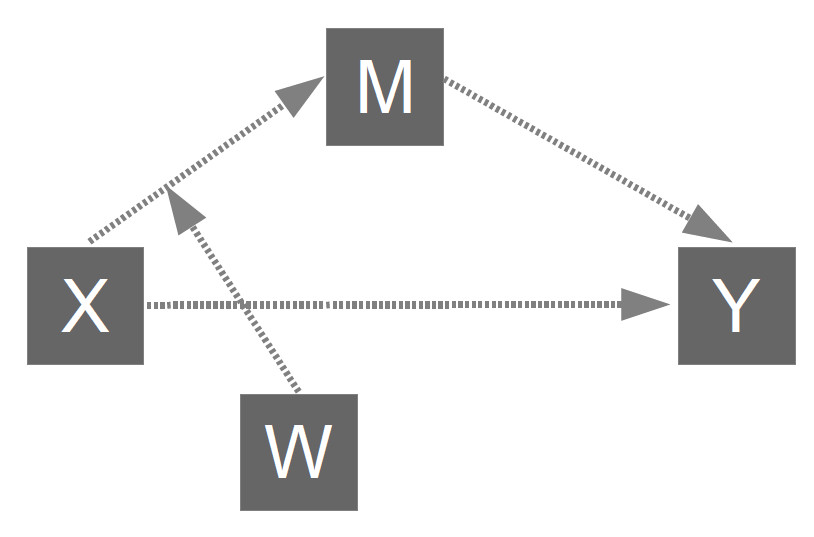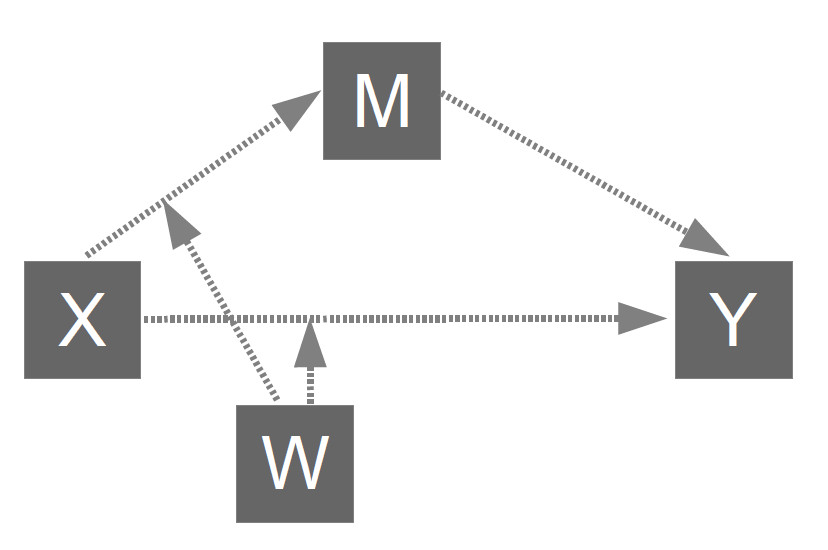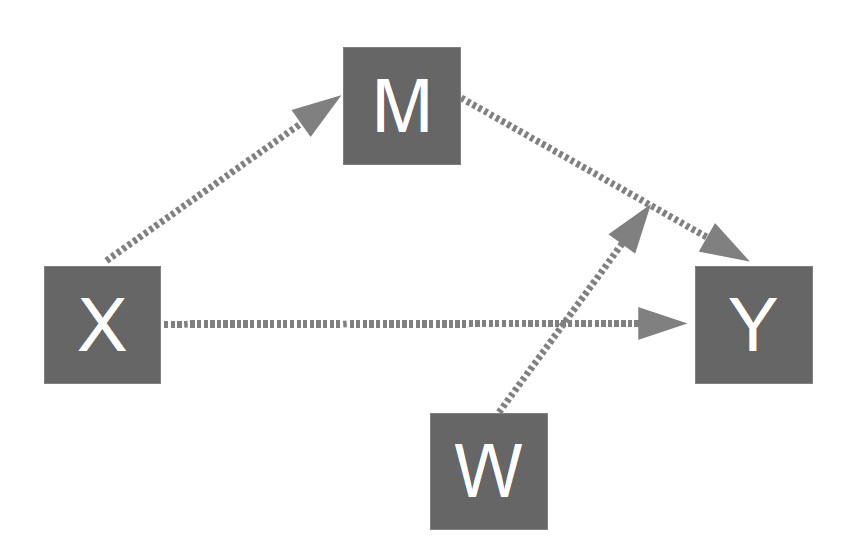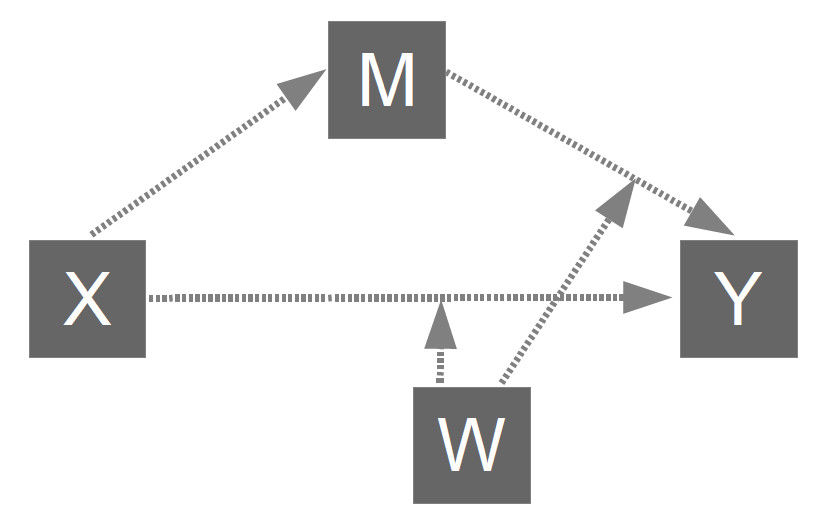﻿ Moderated Mediation with JASP

# Moderated Mediation Analysis with JASP

Testing models 7, 8, 14, 15 with the JASP statistics software

Arndt Regorz, Dipl. Kfm. & M.Sc. Psychologie, 07/21/2021

If you want to test a moderated mediation (=conditional process analysis) with JASP this video tutorial is going to show you how. Based on the JASP's SEM module you will learn how to test one of the four main moderated mediation models: Model 7, model 8, model 14 or model 15 (based on the numbers of Hayes' PROCESS macro).

(Note: When you click on this video you are using a service offered by YouTube.)

Primarily, the video will explain how to implement PROCESS model 8 in JASP, that is a moderated mediation (conditional process analysis) with a moderated a-path from the independent variable to the mediator and a moderated direct effect c'. On this companion webpage to that video you can find the necessary code for all four models (7, 8, 14, 15).

In all syntax examples I have used the following variable names:

• IV = independent variable
• DV = dependent variable
• MED = mediator
• MOD = moderator
• INT_AC = interaction of IV and MOD (for a moderation of the a-path and/or of the c'-path)
• INT_B = interaction of MED and MOD (for a moderation of the b-path)

The code tries to replicate the results you would get running the PROCESS macro in SPSS or R. However, due to differences in the bootstrapping procedure you will not get the exact same confidence intervals.

## Disclaimer

No warranty: I make no warranties of any kind concerning the functionality or correctness of the code on this page.

## 1. Model 7 (moderated a-path)To test this model you can use the following lavaan syntax:

#regression a-path
MED ~ a1 * IV + a2* MOD + a3 * INT_AC

#regression b-path and c'-path
DV ~ c1 * IV + b1* MED

#index of moderated mediation
IMM := a3 * b1

If you get a significant index of moderated mediation (= confidence interval does not include zero), you can test conditional indirect effects with these additional lines:

#conditional indirect effects

CIE_L := (a1 + a3 * (MEAN-SD)) * b1
CIE_M :=(a1 + a3 * MEAN) * b1
CIE_H := (a1 + a3 * (MEAN+SD)) * b1

For 'MEAN' and 'SD' you have to put in the values for mean and standard deviation of you moderator variable.

Please don't forget to use the bootstrap option (with 5,000 bootstrap samples or more).

In general, I recommend using model 8 instead of model 7 because in most research situations we do not know in advance whether the direct effect c' is moderated.

## 2. Model 8 (moderated a-path and moderated c'-path)To test this model you can use the following lavaan syntax:

#regression a-path
MED ~ a1 * IV + a2* MOD + a3 * INT_AC

#regression b-path and c'-path
DV ~ c1 * IV + c2 * MOD + c3 * INT_AC + b1* MED

#index of moderated mediation
IMM := a3 * b1

If you get a significant index of moderated mediation (= confidence interval does not include zero), you can test conditional indirect effects with these additional lines:

#conditional indirect effects

CIE_L := (a1 + a3 * (MEAN-SD)) * b1
CIE_M :=(a1 + a3 * MEAN) * b1
CIE_H := (a1 + a3 * (MEAN+SD)) * b1

For 'MEAN' and 'SD' you have to put in the values for mean and standard deviation of you moderator variable.

Please don't forget to use the bootstrap option (with 5,000 bootstrap samples or more).

## 3. Model 14 (moderated b-path)To test this model you can use the following lavaan syntax:

#regression a-path
MED ~ a1 * IV

#regression b-path and c'-path
DV ~ c1 * IV + b1* MED + b2 * MOD + b3 * INT_B

#index of moderated mediation
IMM := a1 * b3

If you get a significant index of moderated mediation (= confidence interval does not include zero), you can test conditional indirect effects with these additional lines:

#conditional indirect effects

CIE_L := (b1 + b3 * (MEAN-SD)) * a1
CIE_M :=(b1 + b3 * MEAN) * a1
CIE_H := (b1 + b3 * (MEAN+SD)) * a1

For 'MEAN' and 'SD' you have to put in the values for mean and standard deviation of you moderator variable.

Please don't forget to use the bootstrap option (with 5,000 bootstrap samples or more).

In general, I recommend using model 15 instead of model 14 because in most research situations we do not know in advance whether the direct effect c' is moderated.

## 4. Model 15 (moderated b-path and moderated c'-path)To test this model you can use the following lavaan syntax:

#regression a-path
MED ~ a1 * IV

#regression b-path and c'-path
DV ~ c1 * IV + c2 * MOD + c3 * INT_AC + b1* MED + b3 * INT_B
# (kein Pfad b2 angegeben, da hier identisch mit c2)

#index of moderated mediation
IMM := a1 * b3

If you get a significant index of moderated mediation (= confidence interval does not include zero), you can test conditional indirect effects with these additional lines:

#conditional indirect effects

CIE_L := (b1 + b3 * (MEAN-SD)) * a1
CIE_M :=(b1 + b3 * MEAN) * a1
CIE_H := (b1 + b3 * (MEAN+SD)) * a1

For 'MEAN' and 'SD' you have to put in the values for mean and standard deviation of you moderator variable.

Please don't forget to use the bootstrap option (with 5,000 bootstrap samples or more).